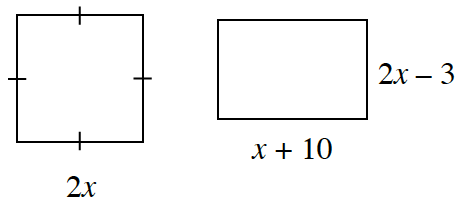### Home > CCG > Chapter 8 > Lesson 8.2.1 > Problem8-76

8-76.

If the rectangles below have the same area, find $x$. Is there more than one answer? Show all work.If the areas are the same, how can you set up an equation to solve for $x$?

Based on this equation, can there be two answers?
Remember with the area of a rectangle being $\text{base}\times\text{height}$, you end up with $\left(2x\right)\left(2x\right)=\left(x+10\right)\left(2x-3\right)$.